# 1.本章学习总结（2分）

## 1.1 思维导图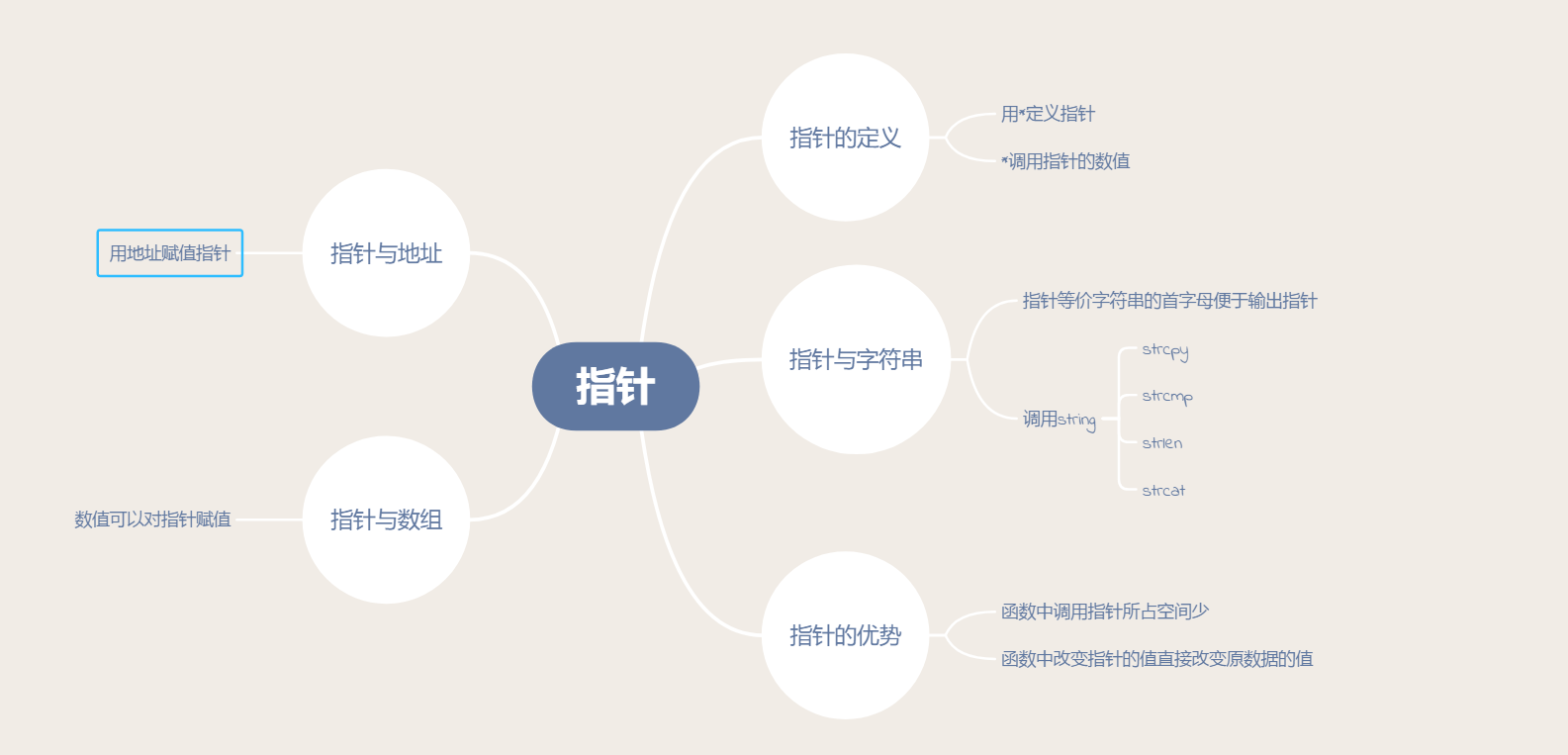## 1.2 本章学习体会及代码量学习体会

### 1.2.1学习体会

学习体会：光阴似箭，转眼间一个月又过去了，学习更加坦然了。这好比下棋，下棋少的人步步惊心很在乎输赢，下棋多的人不断到时觉得不是那么重要了，大概这是一种坦然。打代码总是有解不出来的题，一个人也有状态不好的一天，如果真的要在乎那么多，人生会变得很累。学习体会说说具体的，1.采用我自创的新的学习方法，开始跟上课堂进度了。2.指针数组这块内容不是特别难，只要用心一般都能跟上。3.学习了一些指针的知识，时间还是安排不过来，没有花太多时间复习，原因有几点，第一点是不知道要花多长时间复习，第二点是就算花了时间复习也很容易遗忘，第三点是知识还难以灵活应用，做习题时还是要查书。



### 1.2.2代码量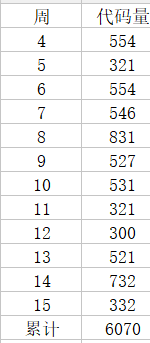## 2.PTA总分

### 2.1截图PTA中题目集的排名得分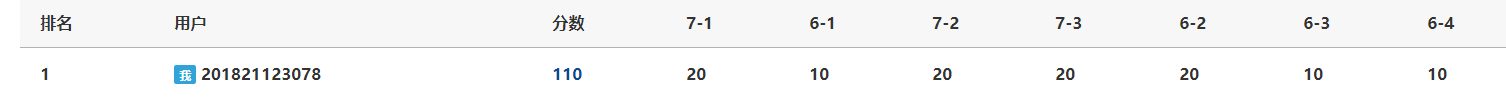## 3.PTA实验作业

### 3.1PTA题目1

给定一句英语，要求编写程序，将句中所有单词的顺序颠倒输出。


### 3.1.1算法分析

void Fun(char*p,int g)
begin
g=g-1
while(1)
while((*(p+g)=='\n')且(g>=0))
g--;
if(g<0)then return;
for(i=g;(*(p+i)!='\n')且(i>=0);i--)//由后往前寻找单词第一个字母的位置
n1=i;
for(j=n1;(*(p+j)!='\n')&&(j<=g);j++)//输出单词
输出*（p+j）
g=i;
if(g<=0)  then return;
while((*(p+g)=='\n')且(g>=0))
g--;
if(g>=0)
输出空格
end while
end

定义字符数组  a;
gets(a);
g=strlen(a);
for i =0 to g-1//除去重复的空格
if a[i]为空格
then  a[i]='\n';
end if
end for
Fun(a,g);
end


### 3.1.2代码截图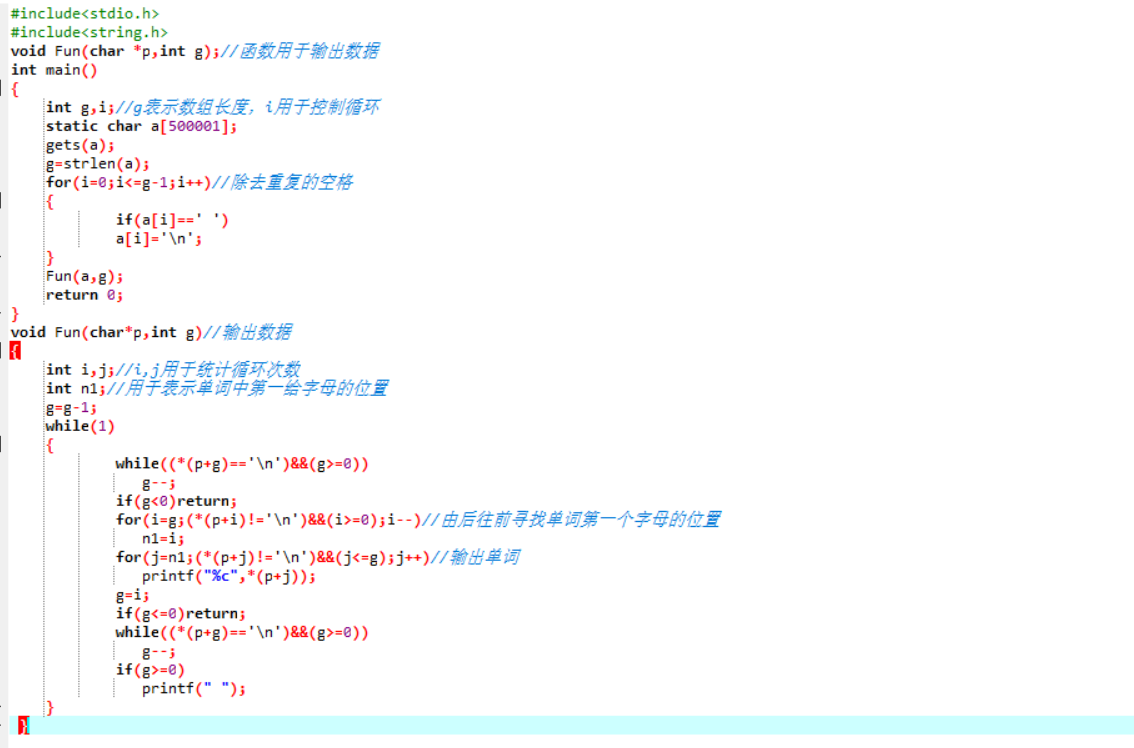### 3.1.3PTA提交列表及说明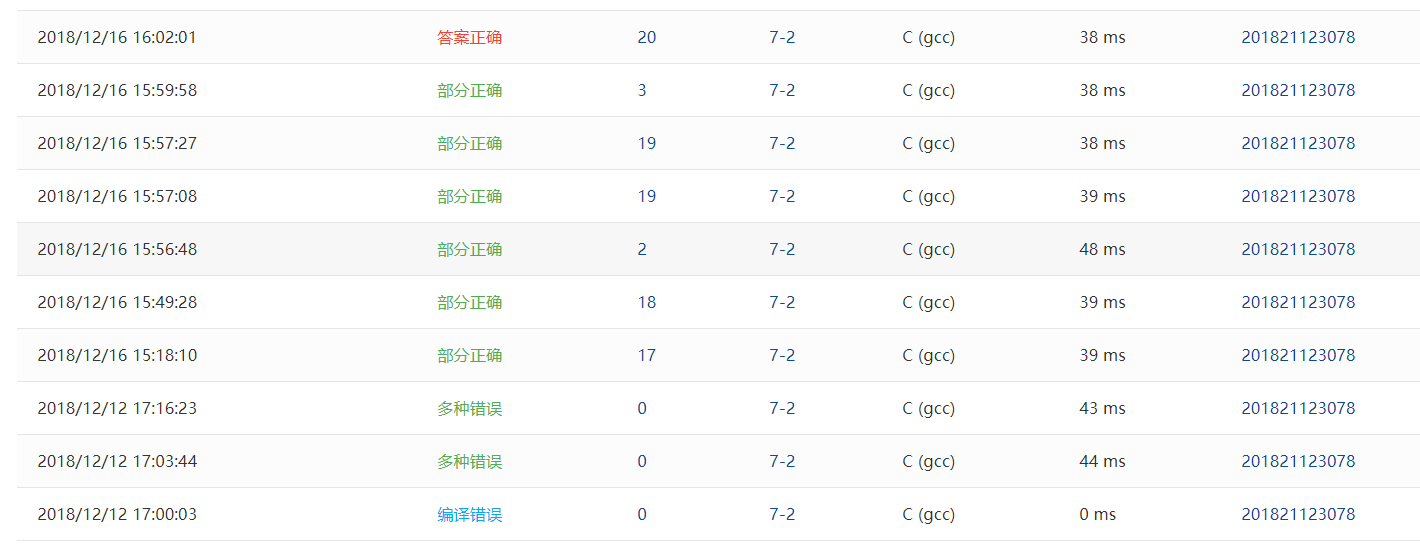Q1:这道题开始时审题不明，没有注意到需要是倒着输出字符串同时不能有重复的空格。
A1：审题清楚后开始作答，有得到一些分数。、
Q2：虽然得到了大部分分数，但是还有三个测试点没过。
A2：是格式错误，最后都会出现空格，这点我修改了条件，在循环语句开始时加入了一个判断，如果数组到了开头，不用输出空格。


## 4.大作业（5分）

### 4.1.改造函数介绍

#### 1.函数1、

int Stage1()
{
定义数组number1
输入题目数
while(1)
{
getchar();
if输入的数据合法 then break
end if
system("cls");
getchar();
输出"输入20000结束游戏，输入10000重新开始游戏\n"
输出“请按正确的格式输入题目数\n"
}
system("cls");  //清屏
if(number==20000)return 0;//输入20000结束游戏
if(number==10000)return 3;//输入10000重新开始
for i=1to number
number1=rand()%10+'0';
number1=rand()%10+'0';
number1='=';
switch(rand()%4)//生成运算
{
case 0:number1='*';result=Result(number1);break;
case 1:
if(number1=='0')

then     number1='/';

else
number1='/';//转换为小数点后两位
r esult=Result(number1);
result=(int)((result+0.005)*100);
result=(double)result/100;
end if
break;
case 2:number1='+';result=Result(number1);break;
case 3:number1='-';result=Result(number1);break;
end  switch
if(number1==0且number1=='/')i--;
else
输出"输入20000结束游戏，输入10000重新开始游戏\n"
if (number1=='/') then printf("如果答案超过两位小数则四舍五入保留两位小数，输入结果\n");
end  if
else printf("请输入结果\n");
printf("%s",number1);
while(1)
if  输入数据合法 then break
end  if
system("cls");
getchar();
printf("输入20000结束游戏，输入10000重新开始游戏\n") ;
printf("请按正确的格式输入结果!\n");
printf("%s",number1);;
end while
if(scanResult==20000)  then  return 0;
else if(scanResult==10000)   then   return 3;
else if(scanResult==result)
if(number1=='/')
then  printf("答案是%.2f\n",result);
else printf("答案是%.0f\n",result);
end if
IfRight();
right++;
else
if(number1=='/')
then printf("答案是%.2f\n",result);
else printf("答案是%.0f\n",result);
end  if
IfWrong();
wrong++;
end if
system("pause");
system("cls");
}
return 1;
}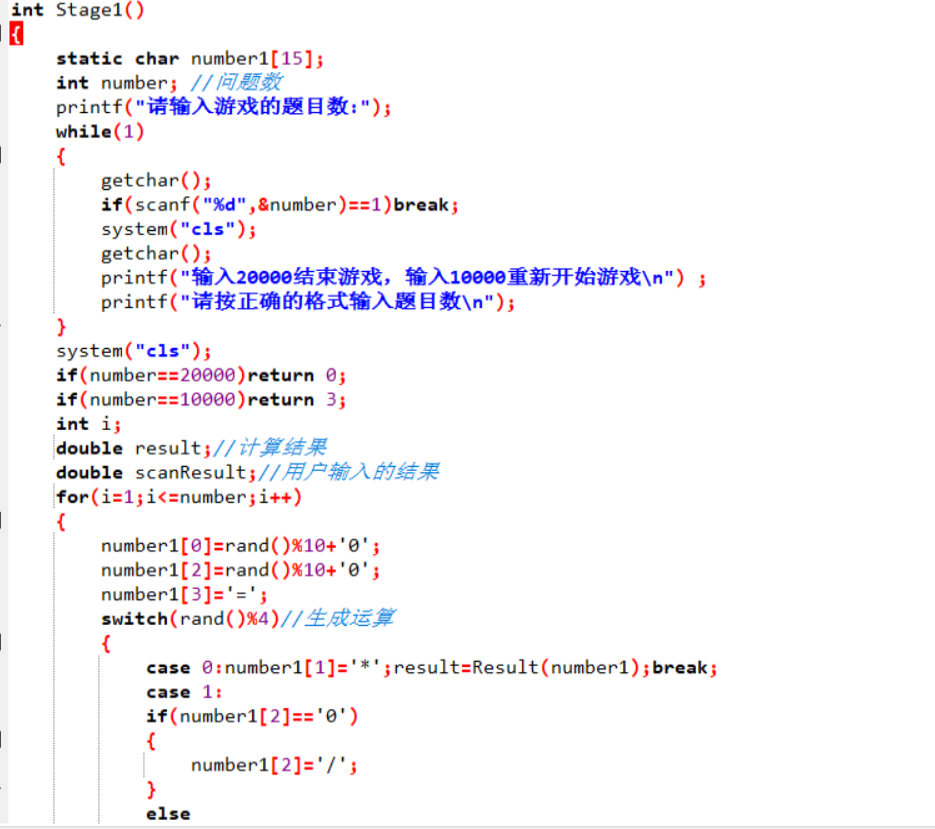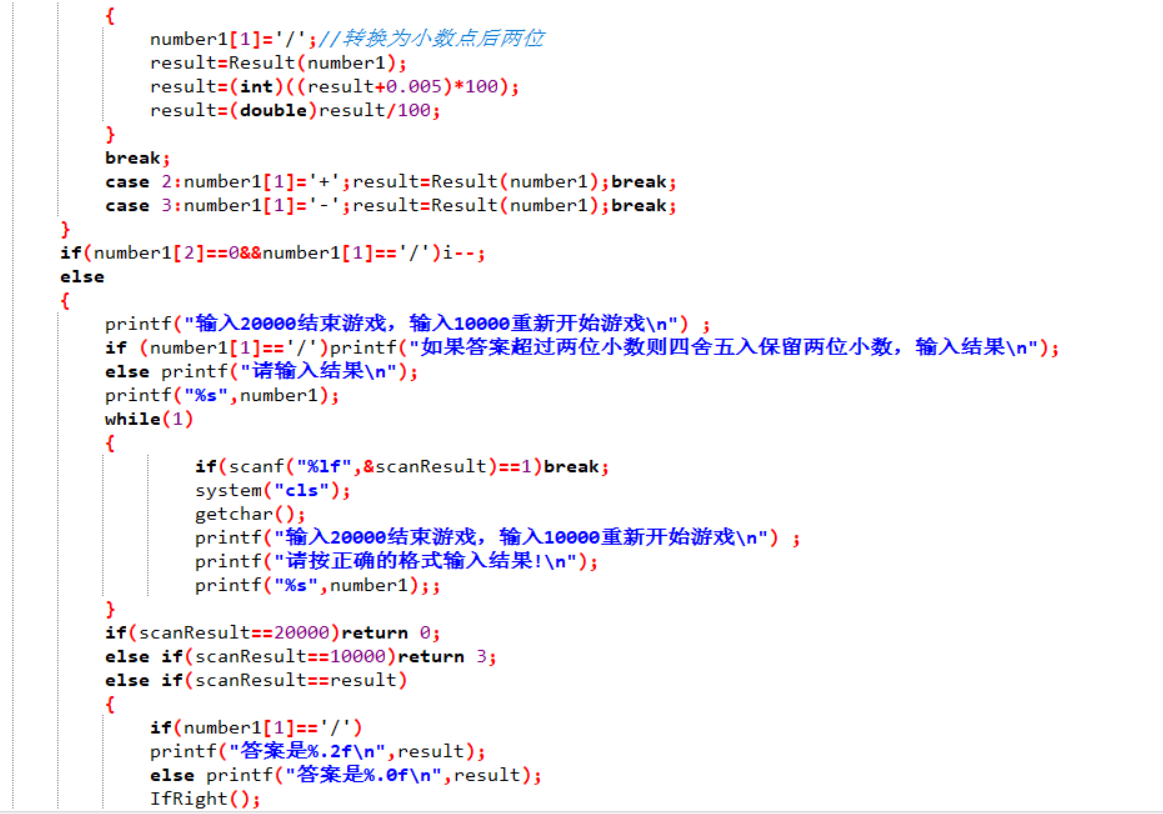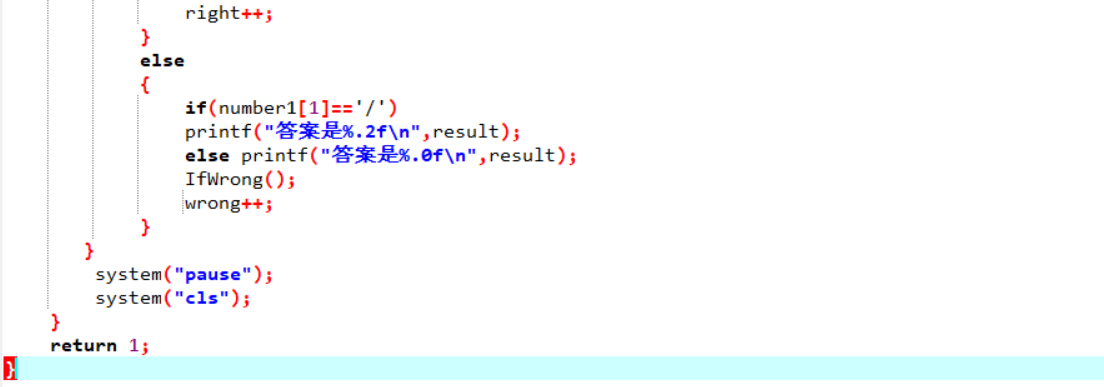#### 2.函数2

double Result(char*p)
begin
for(;*p>='0'&&*p<='9';p++)//转换为十进制数
sum=sum*10+*p-'0';
end for
while(1)
note=*p;//转换符号
if(note=='=')//返回结果
then  return sum;
end  if
p++;
for(;*p>='0'&&*p<='9';p++)
number1=number1*10+*p-'0';
end for
switch(note)//运算
case '+':sum=sum+number1;break;
case '-':sum=sum-number1;break;
case '*':sum=sum*number1;break;
case '/':sum=1.0*sum/number1;break;
end switch
number1=0;//初始化
end while
end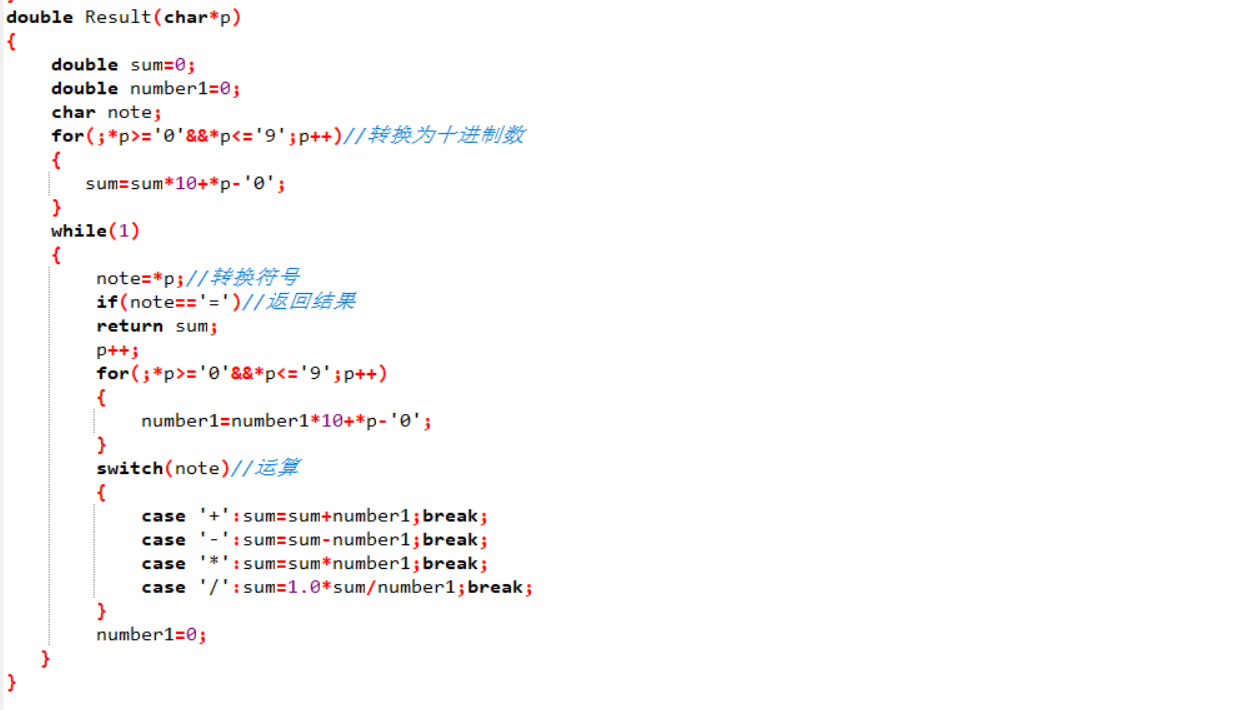#### 3.函数3

int main()
begin
int k;
while(1)
void print();
int stage;//表示难度等级
srand(time(0));//生成随机数

print();
printf("输入等级:");//等级判断
while(scanf("%d",&stage)==0||((stage!=1)&&(stage!=2)&&(stage!=3)&&(stage!=10000)&&(stage!=20000)))
{
system("cls");
getchar();//getchar( )用于吸收系统储存的数据
print();
printf("请按正确的格式输入等级\n");
printf("输入等级:");
}

switch(stage)
{
case 1:k=Stage1();break;
case 2:k=Stage2();break;
case 3:k=Stage3();break;
case 20000:printf("游戏结束");return 0;
case 10000: system("cls"); continue;
}
if(k==0)//对数据进行判断
{
printf("游戏结束");
return 0;
}
if(k==1)
then
CorrectRate();
system("pause");
printf("输入20000结束游戏，输入10000重新开始游戏") ;
while(1)
system("cls");
printf("输入20000结束游戏，输入10000重新开始游戏") ;
printf("\n输入:");
getchar();
scanf("%d",&k);
if(k==10000||k==20000)break;
end if
if(k==20000)
then
printf("游戏结束");
return 0;
end if
if(k==10000)
then
system("cls");
continue;
stage=0;
end  if
end if
if(k==3)
then
system("cls");
right=0;
wrong=0;
continue;
stage=0;//结果清零
end if
end while
return 0;
end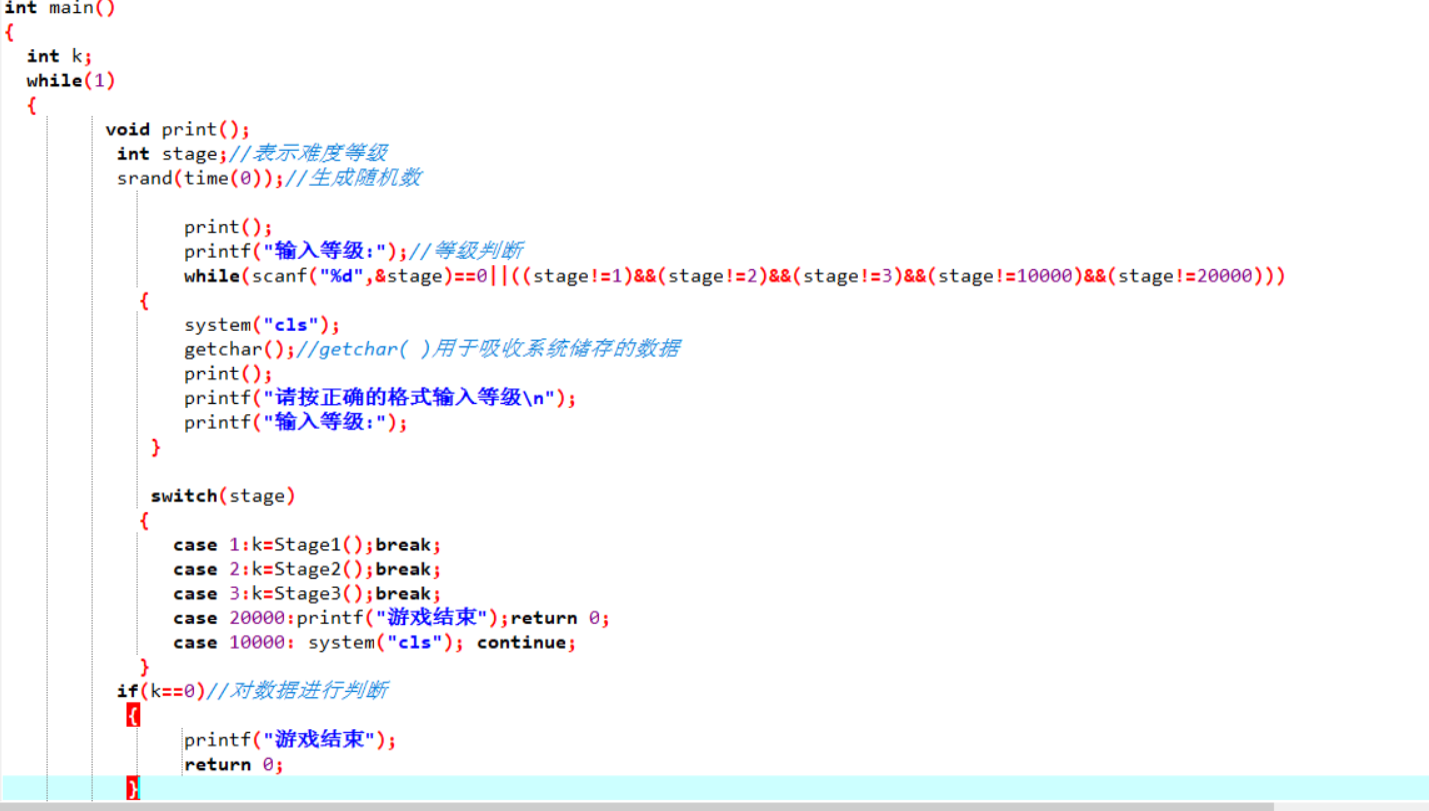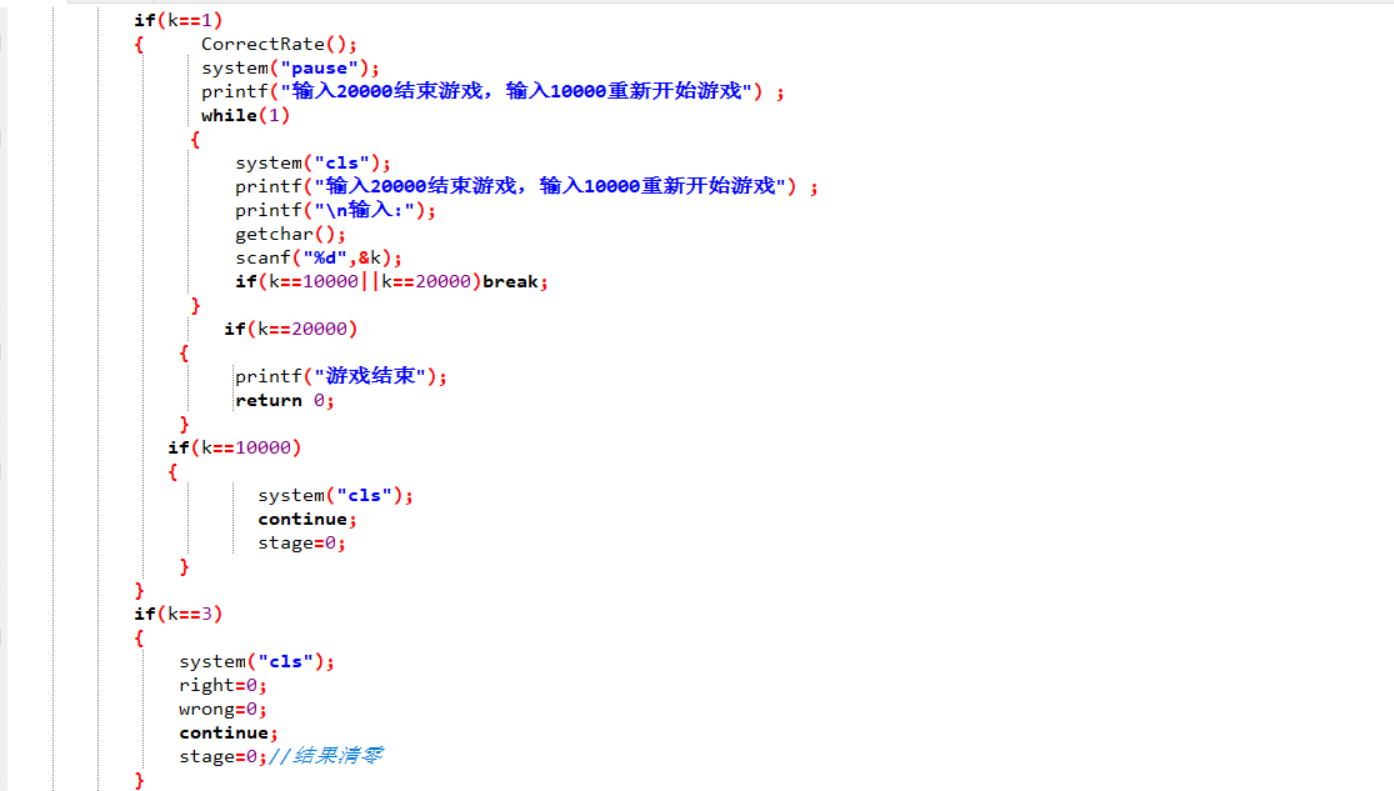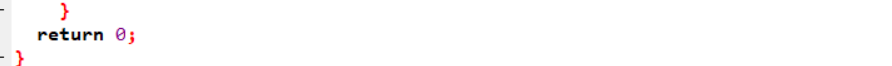#### 4.3 与原有函数代码比较

1.函数1中，原代码采用定义两个数来储存数据，在当前代码中运算，新代码采用数组储存数据，调用函数进行运算。新代码显得更简洁，整个算式更完整，同时运用指针，传输速度快。
2.函数2的变化不大，和原来的代码相比运用了switch函数，整体框架更强。
3.函数3为新增函数，于原来的代码相比数据的完整性更强，可以处理字符型运算。


#### 4.4 改进大作业总结

1.关于审题，我一开始一直不明白代码要怎么改，老师的题目看了很多遍，最后明白需要运用指针，传输字符串，通过字符串运算。
2.在设计新函数的过程中总是出错，发现自己遗忘了一些以前的知识比如switch函数要使用break。还用就是对循环语句及指针的移动把握不准，很容易犯边界错误。
3.时间安排不够，对大作业的完成时间不清楚，导致后面一直赶作业。

posted @ 2018-12-17 16:51  1112+  阅读(181)  评论(1编辑  收藏  举报